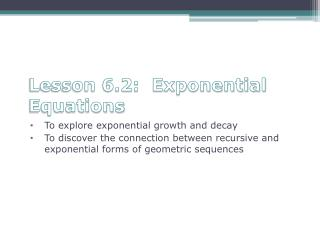DownloadDownload PresentationLesson 6.2: Exponential Equations

# Lesson 6.2: Exponential Equations

Télécharger la présentation## Lesson 6.2: Exponential Equations

- - - - - - - - - - - - - - - - - - - - - - - - - - - E N D - - - - - - - - - - - - - - - - - - - - - - - - - - -
##### Presentation Transcript

1. Lesson 6.2: Exponential Equations To explore exponential growth and decay To discover the connection between recursive and exponential forms of geometric sequences

2. Recursive Routines • Recursive routines are useful for seeing how a sequence develops and for generating the first few terms. • But if you’re looking for the 50th term, you’ll have to do many calculations to find your answer. • In chapter 3, you found that graphs of the points formed a linear pattern, so you learned to write the equation of a line.

3. Recursive Routines • Recursive routines with a constant multiplier create a different pattern. In this lesson you’ll discover the connection between these recursive routines and exponents. • Then with a new type of equation you’ll be able to find any term in a sequence based on a constant multiplier without having to find all the terms before it.

4. Growth of the Koch Curve • In this investigation you will look for patterns in the growth of a fractal. Stage 0

5. Draw Stage 1 figure below the Stage 0 figure. The first segment is drawn for you on the worksheet. Stage 1 should have four segments. Stage 0 Stage 1

6. Describe the curve’s recursive rule so that someone can re-create the curve from your description. Stage 0 Stage 1

7. Using your recursive rule, determine the length of the Stage 1. Record the total length in the chart for Stage 1. Stage 0 Stage 1

8. Draw Stage 2 and 3 for the fractal. Again, the first segment for each stage is drawn for you. Stage 0 Stage 1 Stage 2

9. Record the total length in the chart. Stage 0 Stage 1 Stage 2

10. Find the ratio of the total length at any stage to the total length at the previous stage. • What is the constant multiplier?

11. Use your constant multiplier from the previous step to predict the total length of this fractal at Stages 4 and 5.

12. How many times do you multiply the original length at Stage 0 by the constant multiplier to get the length at Stage 2? Write an expression that calculates the length of Stage 2.

13. How many times do you multiply the original length at Stage 0 by the constant multiplier to get the length at Stage 3? Write an expression that calculates the length of Stage 3.

14. If your expressions in the previous two questions do not use exponents, rewrite them so that they do.

15. Use your constant multiplier from the previous step to predict the total length of this fractal at Stages 5.

16. If x represents the stage number, and y represents the total length of this fractal at any stage, write an equation to model the total length of this fractal at any stage.

17. Create a graph for this equation. • Check the calculator table to see that it contains the same values as your table. • What does the graph tell you about the growth of the Koch curve?

18. Total length Stage Number Constant multiplier Starting length This type of equation is called an exponential equation.

19. The standard form of an exponential equation is

20. Exponential Form Expanded Form

21. Example • Seth deposits \$200 in a savings account. The account pays 5% annual interest. Assuming that he makes no more deposits and no withdrawals, calculate his new balance after 10 years. • Determine the constant multiplier. • Write an equation that can be used to calculate the yearly total.

22. After 10 years